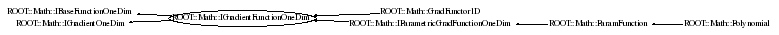```
Documentation for the abstract class IBaseFunctionMultiDim.
Interface (abstract class) for generic functions objects of multi-dimension
Provides a method to evaluate the function given a vector of coordinate values,
by implementing operator() (const double *).
In addition it defines the interface for copying functions via the pure virtual method Clone()
and the interface for getting the function dimension via the NDim() method.
Derived classes must implement the pure private virtual method DoEval(const double *) for the
function evaluation in addition to NDim() and Clone().

@ingroup  CppFunctions

```

## Function Members (Methods)

This is an abstract class, constructors will not be documented.
Look at the header to check for available constructors.

public:
 virtual ~IGradientFunctionOneDim() virtual ROOT::Math::IBaseFunctionOneDim* ROOT::Math::IBaseFunctionOneDim::Clone() const double ROOT::Math::IGradientOneDim::Derivative(double x) const double ROOT::Math::IGradientOneDim::Derivative(const double* x) const virtual void FdF(double x, double& f, double& df) const void ROOT::Math::IGradientOneDim::Gradient(const double* x, double* g) const double ROOT::Math::IBaseFunctionOneDim::operator()(double x) const double ROOT::Math::IBaseFunctionOneDim::operator()(const double* x) const ROOT::Math::IGradientFunctionOneDim& operator=(const ROOT::Math::IGradientFunctionOneDim&)

## Class Charts## Function documentation

void FdF(double x, double& f, double& df) const
```Optimized method to evaluate at the same time the function value and derivative at a point x.
Often both value and derivatives are needed and it is often more efficient to compute them at the same time.
Derived class should implement this method if performances play an important role and if it is faster to
valuate value and derivative at the same tim

```
```Virtual Destructor (no operations)
`{}`